Tamilnadu State Board New Syllabus Samacheer Kalvi 12th Business Maths Guide Pdf Chapter 3 Integral Calculus II Ex 3.2 Text Book Back Questions and Answers, Notes.

## Tamilnadu Samacheer Kalvi 12th Business Maths Solutions Chapter 3 Integral Calculus II Ex 3.2

Question 1.
The cost of an overhaul of an engine is Rs 10,000 The Operating cost per hour is at the rate of 2x-240 where the engine has run x km. find out the total cost of the engine run for 300 hours after overhaul.
Solution:
Given that the overhaul cost is Rs. 10,000.
The marginal cost is 2x – 240
MC = 2x – 240
C = ∫ MC dx + k
C = x2 – 240x + k
k is the overhaul cost
⇒ k = 10,000
So C = x2 – 240x + 10,000
When x = 300 hours, total cost is
C = (300)2 – 240(300) + 10,000
⇒ C = 90,000 – 72000 + 10,000
⇒ C = 28,000
So the total cost of the engine run for 300 hours after the overhaul is ₹ 28,000.

Question 2.
Elasticity of a function $$\frac { Ey }{Ex}$$ is given by $$\frac { Ey }{Ex}$$ = $$\frac { -7x }{(1-2x)(2+3x)}$$. Find the function when x = 2, y = $$\frac { 3 }{8}$$
Solution: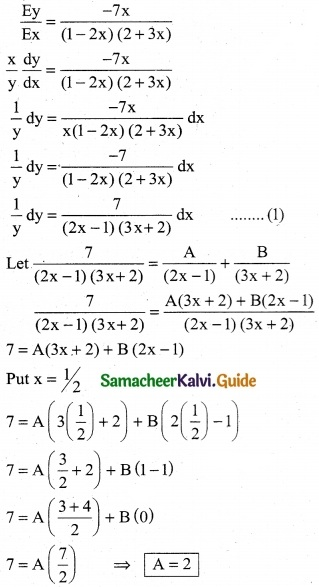Put x = 0
7 = A (3(0) + 2) + B (2(0) – 1)
7 = A (2) + B (-1)
7 = (2) (2) – B
B = 4 – 7
B = -3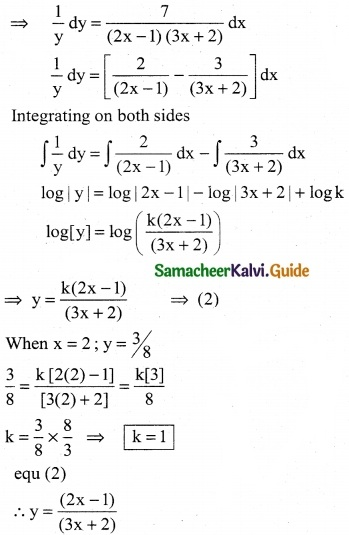Question 3.
The Elasticity of demand with respect to price for a commodity is given by where $$\frac { (4-x)}{x}$$ p is the price when demand is x. find the demand function when the price is 4 and the demand is 2. Also, find the revenue function
Solution:
The elasticity at the demandIntegrating on both sides
∫$$\frac { 1}{(x-4)}$$ = ∫$$\frac { 1}{p}$$ dp
log |x – 4| = log |p| + log k
log |x – 4| = log |pk| ⇒ (x – 4) = pk ……… (1)
when p = 4 and x = 2
(2 – 4) = 4k ⇒ -2 = 4k
k = -1/2
Eqn (1) ⇒ (x – 4) = p(-1/2)
-2 (x – 4) = p ⇒ p = 8 – 2x
Revenue function R = px = (8 – 2x)x
R = 8x – 2x²

Question 4.
A company receives a shipment of 500 scooters every 30 days. From experience it is known that the inventory on hand is related to the number of days x. Since the shipment, I (x) = 500 – 0.03 x², the daily holding cost per scooter is Rs 0.3. Determine the total cost for maintaining inventory for 30 days
Solution:
Here I (x) = 500 – 0.03 x²
C1 = Rs 0.3
T = 30
Total inventory carrying cost
= C1 $$\int _{0}^{T}$$ I(x) dx
= 0.3 $$\int _{0}^{30}$$ (500 – 0.03 x²)dx
= 0.3 [500 x – 0.03($$\frac { x^3 }{3}$$)]$$_{0}^{30}$$
= 0.3 [ 500 x – 0.01 x³]$$_{0}^{30}$$
= 0.3 [500(30) – 0.01 (30)³] – 
= 0.3 [15000 – 0.01 (27000)]
= 0.3 [15000 – 270] = 0.3 
= Rs 4,419Question 5.
An account fetches interest at the rate of 5% per annum compounded continuously an individual deposits Rs 1000 each year in his account. how much will be in the account after 5 years (e0.25 = 1.284)
Solution:
P = 1000
r = $$\frac { 5 }{1000}$$ = 0.05
N = 5
Annuity = $$\int _{0}^{5}$$ 1000 e0.05t dt
= 1000 [ $$\frac { e^{0.05t} }{0.05}$$ ] $$_{0}^{5}$$
= $$\frac { 1000 }{0.05}$$ [e0.05(5) – e0]
= 20000 [e0.25 – 1]
= 20000 [1.284 – 1]
= 20000 [0.284]
= Rs 5680

Question 6.
The marginal cost function of a product is given by $$\frac { dc }{dx}$$ = 100 – 10x + 0.1 x² where x is the output. Obtain the total and average cost function of the firm under the assumption, that its fixed cost is t 500
Solution:
$$\frac { dc }{dx}$$ = 100 – 10x + 0.1 x² and k = Rs 500
dc = (100 – 10x + 0.1 x²) dx
Integrating on both sides,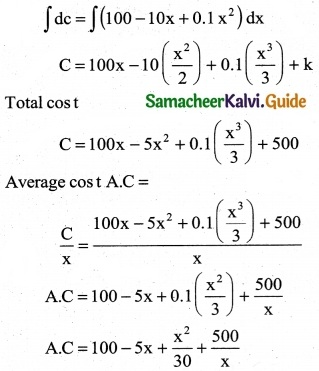Question 7.
The marginal cost function is M.C = 300 x2/5 and the fixed cost is zero. Find the total cost as a function of x
Solution:
M.C = 300 x2/5 and fixed cost K = 0
Total cos t = ∫M.C dx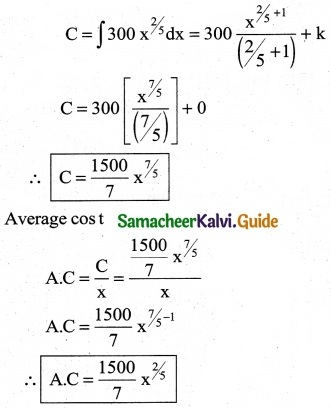Question 8.
If the marginal cost function of x units of output is $$\frac { a }{\sqrt {ax+b}}$$ and if the cost of output is zero. Find the total cost as a function of x.
Solution: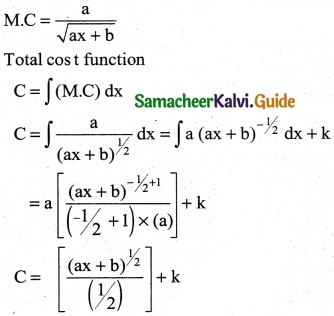∴ C(x) = 2(ax + b)1/2 + k …….. (1)
When x = 0
eqn (1) ⇒ 0 = 2 [a(0) + b]1/2 + k
k = -2(b)1/2 ⇒ k = -2√b
Required cost function
C(x) = 2(ax + b)1/2 – 2√b
∴ C = 2$$\sqrt { ax + b}$$ – 2√bQuestion 9.
Determine the cost of producing 200 air conditioners if the marginal cost (is per unit) is C'(x) = $$\frac { x^2 }{200}$$ + 4
Solution: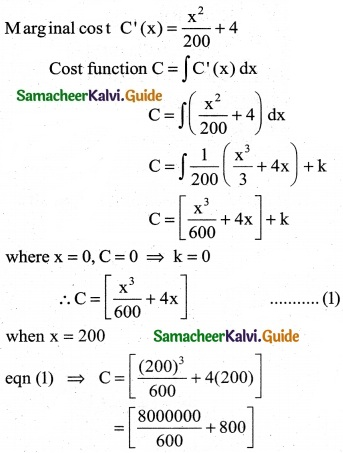= 13333.33 + 800
∴ Cost of producing 200 air conditioners
= Rs 14133.33

Question 10.
The marginal revenue (in thousands of Rupees) function for a particular commodity is 5 + 3 e-0.03x where x denotes the number of units sold. Determine the total revenue from the sale of 100 units (given e-3 = approximately)
Solution:
The marginal Revenue (in thousands of Rupees) function
M.R = 5 + 3-0.03x
Total Revenue from sale of 100 units is
Total Revenue T.R = $$\int _{0}^{ 100}$$ M.R dx= [500 – 100 e-3] – [0 – 100 e°]
= [500 -100 (0.05)] – [-100 (1)]
= [500 – 5]+ 100
= 495 + 100 = 595 thousands
= 595 × 1000
∴ Revenue R = Rs 595000Question 11.
If the marginal revenue function for a commodity is MR = 9 – 4x². Find the demand function.
Solution:
Marginal Revenue function MR = 9 – 4x²
Revenue function R = ∫MR dx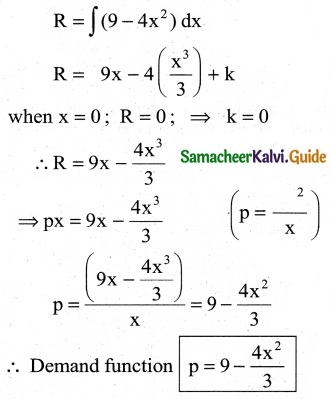Question 12.
Given marginal revenue function $$\frac { 4 }{(2x+3)^2}$$ -1, show that the average revenue function is P = $$\frac { 4 }{6x+9}$$ -1
Solution:
M.R = $$\frac { 4 }{(2x+3)^2}$$ -1
Total Revenue R = ∫M.R dxQuestion 13.
A firms marginal revenue functions is M.R = 20 e-x/10 Find the corresponding demand function.
Solution: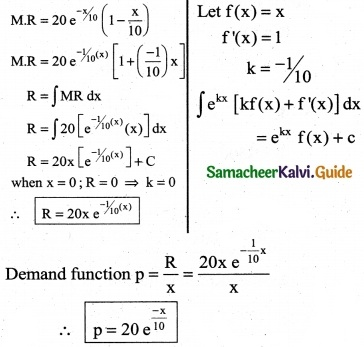Question 14.
The marginal cost of production of a firm is given by C’ (x) = 5 + 0.13 x, the marginal revenue is given by R’ (x) = 18 and the fixed cost is Rs 120. Find the profit function.
Solution:
MC = C'(x) = 5 + 0.13x
C(x) = ∫C'(x) dx + k1
= ∫(5 + 0.13x) dx + k1
= 5x + $$\frac{0.13}{2} x^{2}$$ + k1
When quantity produced is zero, fixed cost is 120
(i.e) When x = 0, C = 120 ⇒ k1 = 120
Cost function is 5x + 0.065x2 + 120
Now given MR = R'(x) = 18
R(x) = ∫18 dx + k2 = 18x + k2
When x = 0, R = 0 ⇒ k2 = 0
Revenue = 18x
Profit P = Total Revenue – Total cost = 18x – (5x + 0.065x2 + 120)
Profit function = 13x – 0.065x2 – 120Question 15.
If the marginal revenue function is R'(x) = 1500 – 4x – 3x². Find the revenue function and average revenue function.
Solution:
Given marginal revenue function
MR = R’(x)= 1500 – 4x – 3x2
Revenue function R(x) = ∫R'(x) dx + c
R = ∫(1500 – 4x – 3x2) dx + c
R = 1500x – 2x2 – x3 + c
When x = 0, R = 0 ⇒ c = 0
So R = 1500x – 2x2 – x3
Average revenue function P = $$\frac{R}{x}$$ ⇒ 1500 – 2x – x2

Question 16.
Find the revenue function and the demand function if the marginal revenue for x units MR = 10 + 3x – x
Solution:
The marginal revenue function
MR = 10 + 3x – x²
The Revenue function
R = ∫(MR) dx
= ∫(10 + 3x – x²)dx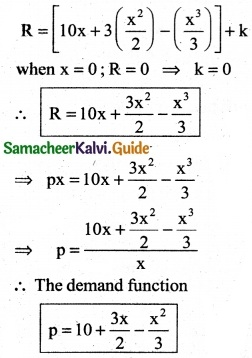Question 17.
The marginal cost function of a commodity is given by Mc = $$\frac { 14000 }{\sqrt{7x+4}}$$ and the fixed cost is Rs 18,000. Find the total cost average cost.
Solution:
The marginal cost function of a commodity
Mc = $$\frac { 14000 }{\sqrt{7x+4}}$$ = 14000 (7x + 4)-1/2
Fixed cost k = Rs 18,000
Total cost function C = ∫(M.C) dx
= ∫14000 (7x + 4)-1/2 dxQuestion 18.
If the marginal cost (MC) of production of the company is directly proportional to the number of units (x) produced, then find the total cost function, when the fixed cost is Rs 5,000 and the cost of producing 50 units is Rs 5,625.
Solution:
M.C αx
M.C = λx
fixed cost k = Rs 5000
Cost function C = ∫(M.C) dx
= ∫λx dx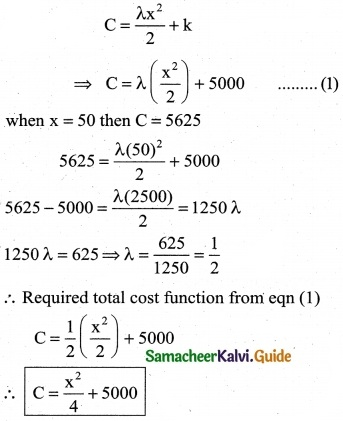Question 19.
If MR = 20 – 5x + 3x², Find total revenue function
Solution:
MR = 20 – 5x + 3x²
Total Revenue function
R = ∫(MR) dx = ∫(20 – 5x + 3x²) dx
R = 20x – $$\frac { 5x^2 }{2}$$ + $$\frac {3x^3 }{3}$$ + k
when x = 0; R = 0 ⇒ k = 0
∴ R = 20x – $$\frac { 5 }{2}$$ x² + x³

Question 20.
If MR = 14 – 6x + 9x², Find the demand function.
Solution:
MR = 14 – 6x + 9x2
R = ∫(14 – 6x + 9x2) dx + k
= 14x – 3x2 + 3x3 + k
Since R = 0, when x = 0, k = 0
So revenue function R = 14x – 3x2 + 3x3
Demand function P = $$\frac{R}{x}$$ = 14 – 3x + 3x2